# Deviance goodness of fit test for Poisson regression

In this post we’ll look at the deviance goodness of fit test for Poisson regression with individual count data. Many software packages provide this test either in the output when fitting a Poisson regression model or can perform it after fitting such a model (e.g. Stata), which may lead researchers and analysts in to relying on it. In this post we’ll see that often the test will not perform as expected, and therefore, I argue, ought to be used with caution.

Poisson regression
In Poisson regression we model a count outcome variable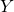as a function of covariates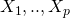. The outcome is assumed to follow a Poisson distribution, and with the usual log link function, the outcome is assumed to have mean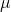, with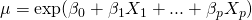Given a sample of data, the parameters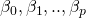are estimated by the method of maximum likelihood.

The deviance
For a fitted Poisson regression the deviance is equal to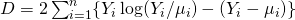where if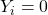, the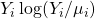term is taken to be zero, and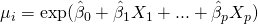denotes the predicted mean for observationbased on the estimated model parameters. The deviance is a measure of how well the model fits the data – if the model fits well, the observed values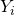will be close to their predicted means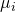, causing both of the terms in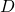to be small, and so the deviance to be small.

The formula for the deviance above can be derived as the profile likelihood ratio test comparing the specified model with the so called saturated model. The saturated model is the model for which the predicted values from the model exactly match the observed outcomes.

Comparing nested models with deviance
The fit of two nested models, one simpler and one more complex, can be compared by comparing their deviances. It turns out that that comparing the deviances is equivalent to a profile log-likelihood ratio test of the hypothesis that the extra parameters in the more complex model are all zero. Because of this equivalence, we can draw upon the result from likelihood theory that as the sample size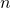becomes large, the difference in the deviances follows a chi-squared distribution under the null hypothesis that the simpler model is correctly specified. The number of degrees of freedom for the chi-squared is given by the difference in the number of parameters in the two models.

The deviance goodness of fit test
Since deviance measures how closely our model’s predictions are to the observed outcomes, we might consider using it as the basis for a goodness of fit test of a given model. While we would hope that our model predictionsare close to the observed outcomes, they will not be identical even if our model is correctly specified – after all, the model is giving us the predicted mean of the Poisson distribution that the observation follows.

To use the deviance as a goodness of fit test we therefore need to work out, supposing that our model is correct, how much variation we would expect in the observed outcomes around their predicted means, under the Poisson assumption. Since the deviance can be derived as the profile likelihood ratio test comparing the current model to the saturated model, likelihood theory would predict that (assuming the model is correctly specified) the deviance follows a chi-squared distribution, with degrees of freedom equal to the difference in the number of parameters. The saturated model can be viewed as a model which uses a distinct parameter for each observation, and so it hasparameters. If our proposed model has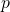parameters, this means comparing the deviance to a chi-squared distribution on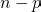parameters.

Performing the deviance goodness of fit test in R
Lets now see how to perform the deviance goodness of fit test in R. First we’ll simulate some simple data, with a uniformally distributed covariate x, and Poisson outcome y:

set.seed(612312)

n <- 1000
x <- runif(n)
mean <- exp(x)
y <- rpois(n,mean)


To fit the Poisson GLM to the data we simply use the glm function:

mod <- glm(y~x, family=poisson)
summary(mod)


which gives as output

Call:
glm(formula = y ~ x, family = poisson)

Deviance Residuals:
Min       1Q   Median       3Q      Max
-2.2547  -0.8859  -0.1532   0.6096   3.0254

Coefficients:
Estimate Std. Error z value Pr(>|z|)
(Intercept) -0.04451    0.05775  -0.771    0.441
x            1.01568    0.08799  11.543   <2e-16 ***
---
Signif. codes:  0 ‘***’ 0.001 ‘**’ 0.01 ‘*’ 0.05 ‘.’ 0.1 ‘ ’ 1

(Dispersion parameter for poisson family taken to be 1)

Null deviance: 1247.7  on 999  degrees of freedom
Residual deviance: 1110.3  on 998  degrees of freedom
AIC: 3140.9

Number of Fisher Scoring iterations: 5


To deviance here is labelled as the ‘residual deviance’ by the glm function, and here is 1110.3. There are 1,000 observations, and our model has two parameters, so the degrees of freedom is 998, given by R as the residual df. To calculate the p-value for the deviance goodness of fit test we simply calculate the probability to the right of the deviance value for the chi-squared distribution on 998 degrees of freedom:

pchisq(mod$deviance, df=mod$df.residual, lower.tail=FALSE)
 0.00733294


The null hypothesis is that our model is correctly specified, and we have strong evidence to reject that hypothesis. So we have strong evidence that our model fits badly. Here we simulated the data, and we in fact know that the model we have fitted is the correct model. So here the deviance goodness of fit test has wrongly indicated that our model is incorrectly specified. But perhaps we were just unlucky – by chance 5% of the time the test will reject even when the null hypothesis is true.

The validity of the deviance goodness of fit test for individual count Poisson data
The asymptotic (large sample) justification for the use of a chi-squared distribution for the likelihood ratio test relies on certain conditions holding. In our setting, we have that the number of parameters in the more complex model (the saturated model) is growing at the same rate as the sample size increases, and this violates one of the conditions needed for the chi-squared justification.

We are thus not guaranteed, even when the sample size is large, that the test will be valid (have the correct type 1 error rate). One of the few places to mention this issue is Venables and Ripley’s book, Modern Applied Statistics with S. Venables and Ripley state that one situation where the chi-squared approximation may be ok is when the individual observations are close to being normally distributed and the link is close to being linear. Pawitan states in his book In All Likelihood that the deviance goodness of fit test is ok for Poisson data provided that the means are not too small. When the mean is large, a Poisson distribution is close to being normal, and the log link is approximately linear, which I presume is why Pawitan’s statement is true (if anyone can shed light on this, please do so in a comment!).

Examining the deviance goodness of fit test for Poisson regression with simulation
To investigate the test’s performance let’s carry out a small simulation study. We will generate 10,000 datasets using the same data generating mechanism as before. For each, we will fit the (correct) Poisson model, and collect the deviance goodness of fit p-values. We will then see how many times it is less than 0.05:

nSim <- 10000
pvalues <- array(0, dim=nSim)

for (i in 1:nSim) {

n <- 1000
x <- runif(n)
mean <- exp(x)
y <- rpois(n,mean)

mod <- glm(y~x, family=poisson)
pvalues[i] <- pchisq(mod$deviance, df=mod$df.residual, lower.tail=FALSE)

}

mean(1*(pvalues<0.05))


The final line creates a vector where each element is one if the p-value is less than 0.05 and zero otherwise, and then calculates the proportion of these which are significant using mean(). When I ran this, I obtained 0.9437, meaning that the deviance test is wrongly indicating our model is incorrectly specified on 94% of occasions, whereas (because the model we are fitting is correct) it should be rejecting only 5% of the time!

To see if the situation changes when the means are larger, let’s modify the simulation. We will now generate the data with Poisson mean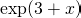, which results in the means ranging from 20 to 55:

nSim <- 10000
pvalues <- array(0, dim=nSim)

for (i in 1:nSim) {

n <- 1000
x <- runif(n)
mean <- exp(3+x)
y <- rpois(n,mean)

mod <- glm(y~x, family=poisson)
pvalues[i] <- pchisq(mod$deviance, df=mod$df.residual, lower.tail=FALSE)

}

mean(1*(pvalues<0.05))


Now the proportion of significant deviance tests reduces to 0.0635, much closer to the nominal 5% type 1 error rate. Thus the claim made by Pawitan appears to be borne out – when the Poisson means are large, the deviance goodness of fit test seems to work as it should.

Conclusion
The above is obviously an extremely limited simulation study, but my take on the results are that while the deviance may give an indication of whether a Poisson model fits well/badly, we should be somewhat wary about using the resulting p-values from the goodness of fit test, particularly if, as is often the case when modelling individual count data, the count outcomes (and so their means) are not large. From my reading, the fact that the deviance test can perform badly when modelling count data with Poisson regression doesn’t seem to be widely acknowledged or recognised.

### 10 thoughts on “Deviance goodness of fit test for Poisson regression”

1.AN EXCELLENT EXAMPLE. HOWEVER, SUPPOSE WE HAVE TWO NESTED POISSON MODELS AND WE WISH TO ESTABLISH IF THE SMALLER OF THE TWO MODELS IS AS GOOD AS THE LARGER ONE. IN THIS SITUATION WHAT WOULD P0.05 MEAN?
MANY THANKS
COLIN(ROMANIA)

•Hi Colin

If you have two nested Poisson models, the deviance can be used to compare the model fits – this is just a likelihood ratio test comparing the two models. If the p-value is significant, there is evidence against the null hypothesis that the extra parameters included in the larger model are zero. That is, there is evidence that the larger model is a better fit to the data then the smaller one.

Jonathan

2.Any updates on this apparent problem?
Thanks,
Dave

•Thanks Dave. I don’t have any updates on the deviance test itself in this setting – I believe it should not in general be relied upon for testing for goodness of fit in Poisson models.

One of the commonest ways in which a Poisson regression may fit poorly is because the Poisson assumption that the conditional variance equals the conditional mean fails. Most commonly, the former is larger than the latter, which is referred to as overdispersion. If overdispersion is present, but the way you have specified the model is correct in so far as how the expectation of Y depends on the covariates, then a simple resolution is to use robust/sandwich standard errors. In this situation the coefficient estimates themselves are still consistent, it is just that the standard errors (and hence p-values and confidence intervals) are wrong, which robust/sandwich standard errors fixes up.

An alternative approach, if you actually want to test for overdispersion, is to fit a negative binomial model to the data. The Poisson model is a special case of the negative binomial, but the latter allows for more variability than the Poisson. The fits of the two models can be compared with a likelihood ratio test, and this is a test of whether there is evidence of overdispersion.

3.What do you think about the Pearson’s Chi-square to test the goodness of fit of a poisson distribution?

4.Very nice post!!!!

5.What if we have an observated value of 0(zero)? How do we calculate the deviance in that particular case?

6.Hello, I am trying to figure out why I’m not getting the same values of the deviance residuals as R, and I be so grateful for any guidance.

Following your example, is this not the vector of predicted values for your model: pred = predict(mod, type=”response”)?

And are these not the deviance residuals: residuals(mod)?

Why then does residuals(mod) not equal 2*y *log( y / pred ) – (y – pred) ?

7.8.